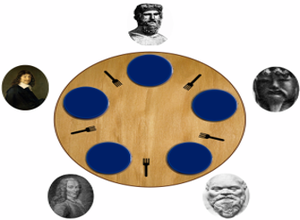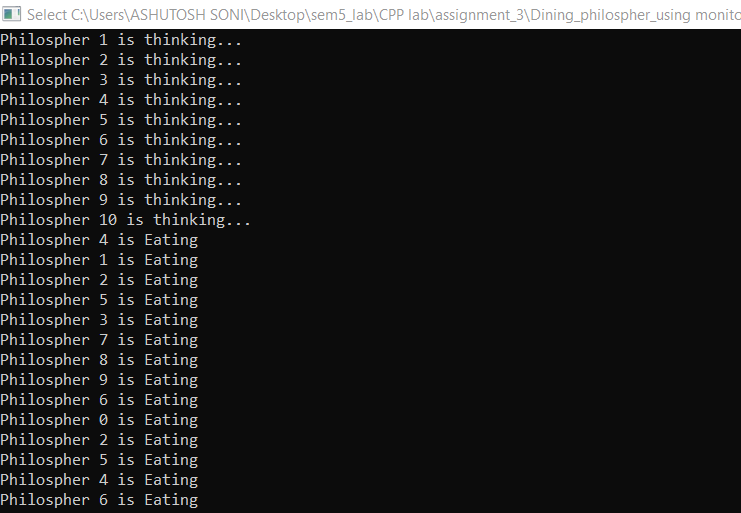Open In App

# Dining Philosophers problem

Overview :
Dining Philosophers Problem States that there are 5 Philosophers who are engaged in two activities Thinking and Eating. Meals are taken communally in a table with five plates and five forks in a cyclic manner as shown in the figure.

Constraints and Condition for the problem :

1. Every Philosopher needs two forks in order to eat.
2. Every Philosopher may pick up the forks on the left or right but only one fork at once.
3. Philosophers only eat when they had two forks. We have to design such a protocol i.e. pre and post protocol which ensures that a philosopher only eats if he or she had two forks.
4. Each fork is either clean or dirty.Solution :
Correctness properties it needs to satisfy are :

1. Mutual Exclusion Principle –
No two Philosophers can have the two forks simultaneously.
Each philosopher can get the chance to eat in a certain finite time.
3. Free from Starvation –When few Philosophers are waiting then one gets a chance to eat in a while.
4. No strict Alternation.
5. Proper utilization of time.

Algorithm(outline) :

```loop forever
p1: think
p2: preprotocol
p3: eat
p4: postprotocol```

First Attempt :
We assume that each philosopher is initialized with its index I and that addition is implicitly modulo 5. Each fork is modeled as a semaphore where wait corresponds to taking a fork and signal corresponds to putting down a fork.

Algorithm –

```semaphore array[0..4] fork ← [1, 1, 1, 1, 1]
loop forever
p1 : think
p2 : wait(fork[i])
p3 : wait(fork[i + 1])
p4 : eat
p5 : signal(fork[i])
p6 : signal(fork[i + 1])```

Problem with this solution :
This solution may lead to a deadlock under an interleaving that has all the philosophers pick up their left forks before any of them tries to pick up a right fork. In this case, all the Philosophers are waiting for the right fork but no one will execute a single instruction.

Second Attempt :
One way to tackle the above situation is to limit the number of philosophers entering the room to four. By doing this one of the philosophers will eventually get both the fork and execute all the instruction leading to no deadlock.

Algorithm –

```semaphore array[0..4] fork ← [1, 1, 1, 1, 1]

semaphore room ← 4
loop forever
p1 : think
p2 : wait(room)
p3 : wait(fork[i])
p4 : wait(fork[i + 1])
p5 : eat
p6 : signal(fork[i])
p7 : signal(fork[i + 1])
p8 : signal(room)```

In this solution, we somehow interfere with the given problem as we allow only four philosophers.

Third Attempt :
We use the asymmetric algorithm in the attempt where the first four philosophers execute the original solution but the fifth philosopher waits for the right fork and then for the left fork.

Algorithm –

`semaphore array [0..4] fork ← [1,1,1,1,1]`

For the first four philosophers –

```loop forever
p1 : think
p2 : wait(fork[i])
p3 : wait(fork[i + 1])
p4 : eat
p5 : signal(fork[i])
p6: signal(fork[i + 1])```

For the fifth philosopher –

```loop forever
p1 : think
p2 : wait(fork)
p3 : wait(fork)
p4 : eat
p5 : signal(fork)
p6 : signal(fork)```

Note –
This solution is also known as Chandy/Mishra Solution.

1. Allows a large degree of concurrency.
2. Free from Starvation.
4. More Flexible Solution.
5. Economical
6. Fairness
7. Boundedness.

The above discussed the solution for the problem using semaphore. Now with monitors, Here, Monitor maintains an array of the fork which counts the number of free forks available to each philosopher. The take Forks operation waits on a condition variable until two forks are available. It decrements the number of forks available to its neighbor before leaving the monitor. After eating, a philosopher calls release Forks which updates the array fork and checks if freeing these forks makes it possible to signal.

Algorithm –

```monitor ForkMonitor:
integer array[0..4]
fork ← [2,2,2,2,2]
condition array[0..4]OKtoEat

operation takeForks(integer i)
if(fork[i]!=2)
waitC(OKtoEat[i])

fork[i+1]<- fork[i+1]-1
fork[i-1] <- fork[i-1]-1

operation releaseForks(integer i)
fork[i+1] <- fork[i+1]+1
fork[i-1] <- fork[i-1]

if(fork[i+1]==2)
signalC(OKtoEat[i+1])

if(fork[i-1]==2)
signalC(OKtoEat[i-1])```

For each Philosopher –

``` loop forever :
p1 : think
p2 : takeForks(i)
p3 : eat
p4 : releaseForks(i)```

Here Monitor will ensure all such needs mentioned above.

Implementation in C++ :
Here is the Program for the same using monitors in C++ as follows.

## C++14

 `//Submitted by : Ashutosh Soni` `// Header file include``#include ``#include ``#include ``using` `namespace` `std;` `#define N 10``#define THINKING 2``#define HUNGRY 1``#define EATING 0``#define LEFT (phnum + 4) % N``#define RIGHT (phnum + 1) % N` `// Philosopher index``int` `phil[N];``int` `times = 200;` `class` `monitor {` `    ``// state of the philosopher``    ``int` `state[N];` `    ``// Philosopher condition variable``    ``pthread_cond_t phcond[N];` `    ``// mutex variable for synchronization``    ``pthread_mutex_t condLock;` `public``:``    ``// Test for the desired condition``    ``// i.e. Left and Right philosopher are not reading``    ``void` `test(``int` `phnum)``    ``{` `        ``if` `(state[(phnum + 1) % 5] != EATING``            ``and state[(phnum + 4) % 5] != EATING``            ``and state[phnum] == HUNGRY) {``            ``state[phnum] = EATING;` `            ``pthread_cond_signal(&phcond[phnum]);``        ``}``    ``}` `    ``// Take Fork function``    ``void` `take_fork(``int` `phnum)``    ``{` `        ``pthread_mutex_lock(&condLock);` `        ``// Indicates it is hungry``        ``state[phnum] = HUNGRY;` `        ``// test for condition``        ``test(phnum);` `        ``// If unable to eat.. wait for the signal``        ``if` `(state[phnum] != EATING) {``            ``pthread_cond_wait(&phcond[phnum], &condLock);``        ``}``        ``cout << ``"Philosopher "` `<< phnum << ``" is Eating"``             ``<< endl;` `        ``pthread_mutex_unlock(&condLock);``    ``}` `    ``// Put Fork function``    ``void` `put_fork(``int` `phnum)``    ``{` `        ``pthread_mutex_lock(&condLock);` `        ``// Indicates that I am thinking``        ``state[phnum] = THINKING;` `        ``test(RIGHT);``        ``test(LEFT);` `        ``pthread_mutex_unlock(&condLock);``    ``}` `    ``// constructor``    ``monitor()``    ``{` `        ``for` `(``int` `i = 0; i < N; i++) {``            ``state[i] = THINKING;``        ``}` `        ``for` `(``int` `i = 0; i < N; i++) {``            ``pthread_cond_init(&phcond[i], NULL);``        ``}` `        ``pthread_mutex_init(&condLock, NULL);``    ``}` `    ``// destructor``    ``~monitor()``    ``{` `        ``for` `(``int` `i = 0; i < N; i++) {``            ``pthread_cond_destroy(&phcond[i]);``        ``}` `        ``pthread_mutex_destroy(&condLock);``    ``}``}` `// Global Object of the monitor``phil_object;` `void``* philosopher(``void``* arg)``{``    ``int` `c = 0;``    ``while` `(c < times) {``        ``int` `i = *(``int``*)arg;``        ``sleep(1);``        ``phil_object.take_fork(i);``        ``sleep(0.5);``        ``phil_object.put_fork(i);``        ``c++;``    ``}``}` `int` `main()``{` `    ``// Declaration...``    ``pthread_t thread_id[N];``    ``pthread_attr_t attr;` `    ``// Initialization...``    ``pthread_attr_init(&attr);``    ``pthread_attr_setdetachstate(&attr,``                                ``PTHREAD_CREATE_JOINABLE);` `    ``for` `(``int` `i = 0; i < N; i++) {``        ``phil[i] = i;``    ``}` `    ``// Creating...``    ``for` `(``int` `i = 0; i < N; i++) {``        ``pthread_create(&thread_id[i], &attr, philosopher,``                       ``&phil[i]);``        ``cout << ``"Philosopher "` `<< i + 1 << ``" is thinking..."``             ``<< endl;``    ``}` `    ``// Joining....``    ``for` `(``int` `i = 0; i < N; i++) {``        ``pthread_join(thread_id[i], NULL);``    ``}` `    ``// Destroying``    ``pthread_attr_destroy(&attr);``    ``pthread_exit(NULL);` `    ``return` `0;``}`

Output :Output for Dining Philosophers problem for the above program